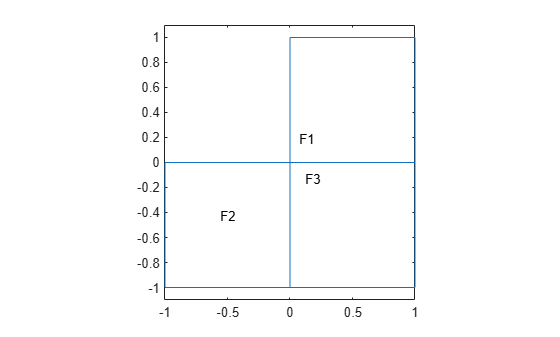# findElectromagneticProperties

Find electromagnetic material properties assigned to geometric region

Since R2021a

## Syntax

``emProperties = findElectromagneticProperties(emagmodel.MaterialProperties,RegionType,RegionID)``

## Description

example

````emProperties = findElectromagneticProperties(emagmodel.MaterialProperties,RegionType,RegionID)` returns the electromagnetic material properties assigned to the specified region of the specified model.```

## Examples

collapse all

Create an electromagnetic model for an electrostatic analysis.

`emagmodel = createpde("electromagnetic","electrostatic");`

Include the L-shaped membrane geometry in the model and plot it with the face labels.

```geometryFromEdges(emagmodel,@lshapeg); pdegplot(emagmodel,"FaceLabels","on") ylim([-1.1 1.1]) axis equal```Specify the vacuum permittivity value in the SI system of units.

`emagmodel.VacuumPermittivity = 8.8541878128E-12;`

Specify a different value of relative permittivity for each face.

```electromagneticProperties(emagmodel,"RelativePermittivity",2.5, ... "Face",1); electromagneticProperties(emagmodel,"RelativePermittivity",2.25, ... "Face",2); electromagneticProperties(emagmodel,"RelativePermittivity",1, ... "Face",3);```

Check the electromagnetic material properties specification for each face.

`findElectromagneticProperties(emagmodel.MaterialProperties,"Face",1)`
```ans = ElectromagneticMaterialAssignment with properties: RegionType: 'Face' RegionID: 1 RelativePermittivity: 2.5000 RelativePermeability: [] Conductivity: [] ```
`findElectromagneticProperties(emagmodel.MaterialProperties,"Face",2)`
```ans = ElectromagneticMaterialAssignment with properties: RegionType: 'Face' RegionID: 2 RelativePermittivity: 2.2500 RelativePermeability: [] Conductivity: [] ```
`findElectromagneticProperties(emagmodel.MaterialProperties,"Face",3)`
```ans = ElectromagneticMaterialAssignment with properties: RegionType: 'Face' RegionID: 3 RelativePermittivity: 1 RelativePermeability: [] Conductivity: [] ```

Create and plot a geometry consisting of two nested spheres.

```gm = multisphere([5 15]); pdegplot(gm,"CellLabels","on","FaceAlpha",0.3)```Create an electromagnetic model for magnetostatic analysis.

`emagmodel = createpde("electromagnetic","magnetostatic");`

Include the geometry in the model.

`emagmodel.Geometry = gm;`

Specify the vacuum permeability value in the SI system of units.

`emagmodel.VacuumPermeability = 1.2566370614E-6;`

Specify a different value of relative permittivity for each cell.

```electromagneticProperties(emagmodel,"RelativePermittivity",2.5, ... "Cell",1); electromagneticProperties(emagmodel,"RelativePermittivity",2.25, ... "Cell",2);```

Check the electromagnetic material properties specification for each cell.

`findElectromagneticProperties(emagmodel.MaterialProperties,"Cell",1)`
```ans = ElectromagneticMaterialAssignment with properties: RegionType: 'Cell' RegionID: 1 RelativePermittivity: 2.5000 RelativePermeability: [] Conductivity: [] ```
`findElectromagneticProperties(emagmodel.MaterialProperties,"Cell",2)`
```ans = ElectromagneticMaterialAssignment with properties: RegionType: 'Cell' RegionID: 2 RelativePermittivity: 2.2500 RelativePermeability: [] Conductivity: [] ```

## Input Arguments

collapse all

Material properties of an electromagnetic model, specified as the `MaterialProperties` property of the model.

Example: `emagmodel.MaterialProperties`

Geometric region type, specified as `"Face"` for a 2-D geometry or `"Cell"` for a 3-D geometry.

Data Types: `char` | `string`

Region ID, specified as a vector of positive integers. Find the face or cell IDs by using `pdegplot` with the `"FaceLabels"` or `"CellLabels"` name-value argument set to `"on"`.

Data Types: `double`

## Output Arguments

collapse all

Material properties assignment, returned as an `ElectromagneticMaterialAssignment` object. For more information, see ElectromagneticMaterialAssignment Properties.

## Version History

Introduced in R2021a